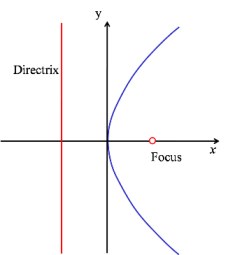The Parabola is a plane curve. The Parabola is a conic section.

The parabola is defined as the path of a point (locus) which moves such that its distance from a fixed point (called the focus) is equal to its distance from a fixed line (called the directrix).

When the directrix is x = -a and the focus is ( a, 0) the parabola will have the equation in Cartesian Coordinates $y^2=4ax$

Rays parallel to the axis of symmetry of a parabolic mirror are reflected through the focus which makes these devices very useful in optical instruments.

Similarly, rays that originate at the focus will produce a parallel beam thereby making parabolic mirrors useful in torches and searchlights.

Show that when the directrix is x = -a and the focus is ( a, 0) then the equation of the curve is $y^2=4ax$